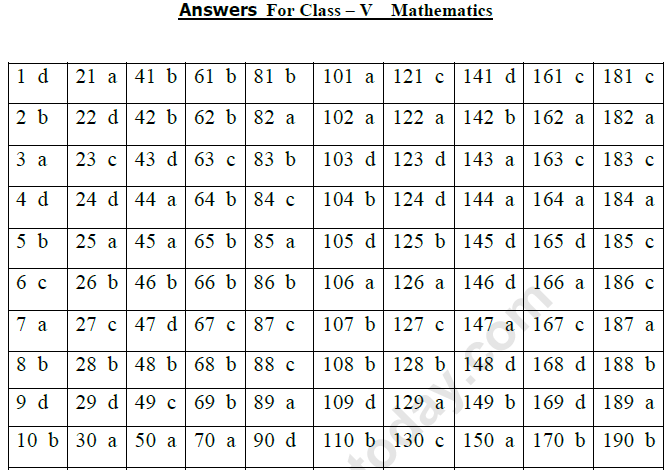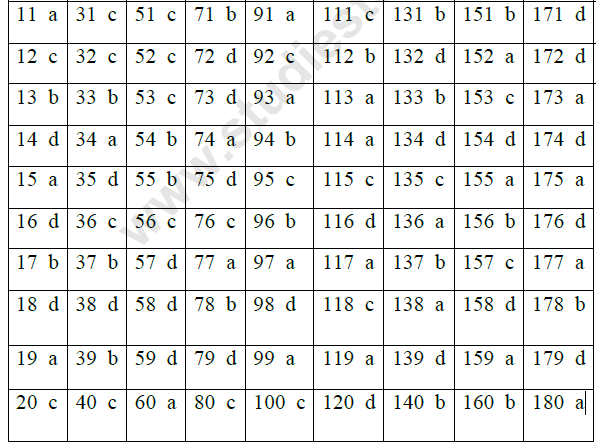# CBSE Class 5 Mathematics MCQs

## MCQ for Class 5 Mathematics Mathematics

Class 5 Mathematics students should refer to the following multiple-choice questions with answers for Mathematics in standard 5. These MCQ questions with answers for Grade 5 Mathematics will come in exams and help you to score good marks

### Mathematics MCQ Questions Class 5 Mathematics with Answers

1) How many times would the digit, 2, be written if you wrote down all the whole numbers from 1 to 100 ?
a) 10 b) 11 c) 19 d) 20

2) The product of the place values of 6 in 438269160, divided by 1000 is
a) 36 b) 3600 c) 60 d) 60000

3) 625 ÷ ____ = 25
a) 25 b) 625 c) 1 d) 250

4) ________ tens make a crore.
a) 1,00,000 b) 1,00,00,000 c) 1,000 d) 10,00,000

5) (10,000 ÷ 50) X 5 = ______
a) 100 b) 1000 c) 2000 d) 40

6) Numeral for Eighty crore eighty lakh eighty one is
a) 80,80,081 b) 80,08,881 c) 80,80,00,081 d) 80,80,08,081

7) 3,00,000 ÷ ________ = 300
a) 1000 b) 3000 c) 100 d) 10,000

8) The difference between the predecessor and the successor of one million is _____
a) 1 b) 2 c) 1,000,000 d) 1,000,001

9) What should be subtracted from the greatest 7 digit number to get 65,43,210 ?
a) 12,34,567 b) 99,99,999 c) 12,34,560 d) 34,56,789

10) 10,00,00,000 – 100 = ___________
a) 99,99,99,900 b) 9,99,99,900 c) 1,00,00,900 d) 10,00,00,900

11) (321 X 9) - ______ = 2,888
a) 1 b) 0 c) 2 d) 3

12) 111 X 111 = _________
a) 1,11,111 b) 12,312 c) 12,321 d) 12,121

13) To get a quotient of 700 and a remainder 48 what number should be divided by 100?
a) 748 b) 70,048 c) 70,000 d) 7,048

14) The largest 9 digit number ending with 7 is
a) 77,77,77,777 b) 10,00,00,007 c) 99,99,99,999 d) 99,99,99,997

15) 25 lakhs + 6 thousands + 4 tens = _________
a) 25,06,040 b) 2,56,00,040 c) 25,640 d) 25, 60, 040

16) The successor of 93,99,999 is _______________
a) 93,91,000 b) 93,99,998 c) 94,99,999 d) 94,00,000

17) 87,88,989 87,78,979 __________ 87,58, 959 The missing number in the above pattern is
a) 87,68,959 b) 87,68,969 c) 87,88,989 d) 87,88,969

18) The digit immediate to the right of ten lakhs shows _______ place.
a) crore b) ten crore c) ten thousand d) lakh

19) The predecessor of 1 crore is __________________
a) 99,99,999 b) 10,01,000 c) 9,99,999 d) 99,99,99,999

20) __________ X 1,000 = 9,98,20,000
a) 9,98,200 b) 9,982 c) 99,820 d) 99,82,000

21) 1 X 2 X 3 X 4 X 5 X 6 = __________
a) 720 b) 21 c) 600 d) 300

22) 7,30,485 + 2,69,514 = ____________
a) 9,99,99, 999 b) 99,999 c) 10,00,009 d) 9,99,999

23) (33 X 3) + (3 ÷ 3) = __________
a) 98 b) 99 c) 100 d) 33

24) The product of two numbers is 10,800. If one number is 90 what is the other number?
a) 10,890 b) 10,710 c) 9,72,000 d) 120

25) 1,00,00,000 – 10,00,000 = __________
a) 90,00,000 b) 9,00,000 c) 9,00,00,000 d) 90,000

26) In which of the following, the numbers are arranged in ascending order?
a) 57071 50371 53071 57301
b) 50371 53071 57071 57301
c) 57301 53071 57071 50371
d) 50371 53071 57301 57071

27) Numbers which are divisible by both 3 and 4 in the following are:
a) 1312 and 2612 b) 4449 and 8804 c) 9924 and 1536 d) 2700 and 3700

27) Which is the odd one in the following?
a) 37 b) 47 c) 57 d) 97

28) The two prime factors of 65 are ____ and _____
a) 1 and 65 b) 5 and 13 c) 60 and 5 d) 15 and 15

29) The 12th multiple of 16 is ________
a) 182 b) 1216 c) 1129 d) 192

30) The least multiple which is common for both 15 and 25 is ______
a) 75 b) 25 c) 50 d) 15

31) If we divide the sum of 45 and 90 by their difference, the answer is
a) neither prime nor composite b) the least prime number
c) the least odd prime number d) the least composite number

32) I am a number between 660 and 665. I am divisible by 3 but not by 6. What number am I ?
a) 661 b) 662 c) 663 d) 664

33) I am a prime number. If you subtract 1 from me, I will become divisible by 9. Who am I ?
a) 29 b) 19 c) 17 d) 11

34) The three numbers whose LCM is 75 are _______, _______ and _______.
a) 5, 15, 25 b) 5, 10, 15 c) 3, 5, 15 d) 3, 50, 25

35) The three prime numbers between 10 and 40 are ______, ______ and ______
a) 17, 27, 37 b) 11, 21, 31 c) 13, 23, 33 d) 13, 29, 37

36) LCM of 3, 8 and 9 is
a) 18 b) 27 c) 72 d) 54

37) The largest prime number between 1 and 100 is
a) 89 b) 97 c) 99 d) 93

38) 672 is divisible by
a) 2 and 3 b) 3 and 4 c) 2 and 6 d) all of these

39) The composite number which comes just after 60 is
a) 61 b) 62 c) 63 c) 64

40) There are ______ prime numbers between 40 and 70
a) 11 b) 10 c) 7 d) 9

41) A factor of 91 is
a) 17 b) 13 c) 31 d) none of these

42) Which among the following are co prime numbers?
a) 9 and 21 b) 7 and 17 c) 14 and 35 d) 12 and 33

43) 48949 is divisible by
a) 2 b) 3 c) 4 d) None of these

44) Fill in the missing multiple. 90, 105, ______, 135, 150
a) 120 b) 15 c) 125 d) 130

45) HCF of 90, 96 and 10 is
a) 2 b) 6 c) 10 d) 1

46) LCM of 7, 14, 17 is
a) 34 b) 238 c) 28 d) None of these

47) LCM of 8 and 10 is
a) 8 b) 80 d) 10 d) 40

48) HCF of 16 and 73 is
a) 16 b) 1 c) 73 d) None of these

49) Factors of 93 are
a) 1, 3, 13, 93 b) 1, 2, 23, 93 c) 1, 3, 31, 93 d) None of these

50) Co prime numbers are numbers whose common factor is
a) 1 b) 2 c) 0 d) their product

51) Which among the following are twin primes?
a) 31 and 37 b) 7 and 11 c) 11 and 13 d) 3 and 7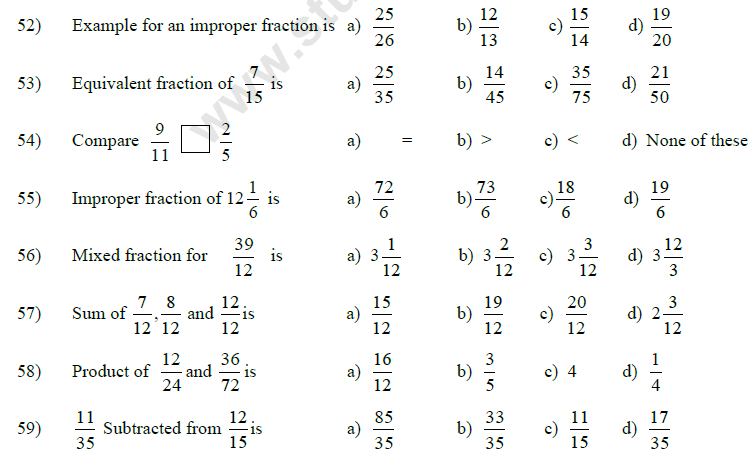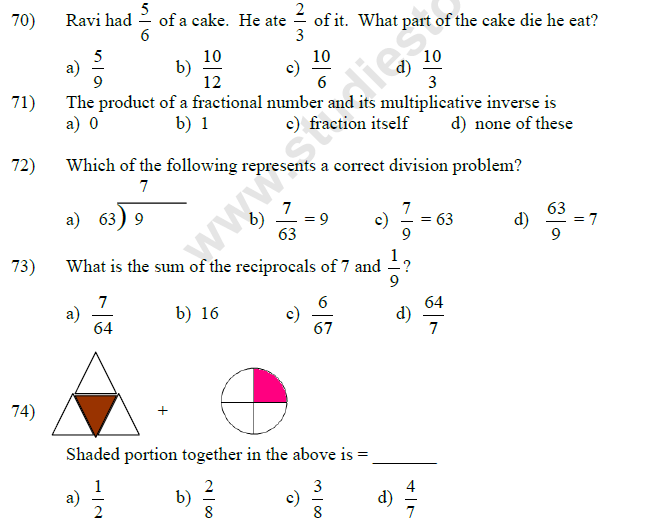75) All fractions that have same value are called ______________ fractions.
a) Like fractions b) Proper fractions c) Unlike fractions d) Equivalent fractions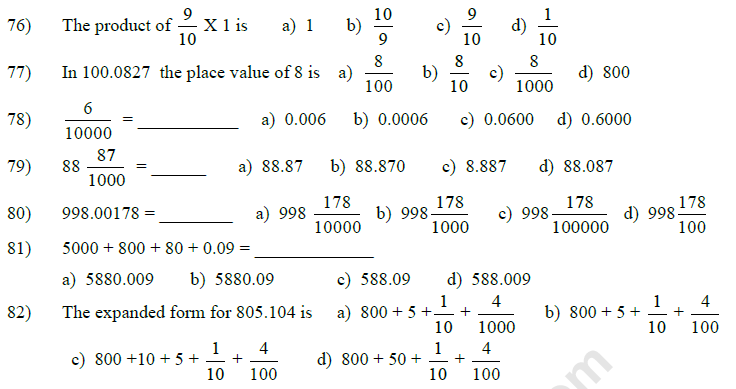83) The like decimal fraction for 5.05, 5.5 is
a) 5.05, 5.05 b) 5.05, 5.50 c) 5.50, 5.50 d) 5.5, 5.5
84) The equivalent decimal fraction for 8.0411 is
a) 8.04101 b) 8.00411 c) 8.04110 d) 8.4110
85) 7.02, 77.02, 6.65 are called as
a) Like decimals b) Equivalent decimals
c) Proper decimals d) Unlike decimals90) I am a decimal number and I am 0.5 less than 1, then I am _______ .

a) 1.5 b) 0.1 c) 0.15 d) 0.5

91) _________ ÷ 1000 = 7.531

a) 7531 b) 75.31 c) 753.1 d) 0.7531

92) Seema had Rs.5 and spent Rs. 2.50 for a pencil. How much money was left with her?

a) Rs. 2.25 b) Rs. 3.50 c) Rs.2.50 d) Rs.7.50

93) 0.7 X 3 = ________

a) 20/10 b) 0.21 c) 7.3 d) 21

94) Ravi covered a distance of 1000 metres in 10 minutes. How much did he cover in 1 minute?

a) 1 km b) 100 m c) 10 km d) 1 m

95) 79.9 X (100.1X 0) = ______

a) 79.900 b) 799.100 c) 0 d) 79.910

96) 65.01 = 65.01 ÷ _______

a) 100 b) 1 c) 10 d) 100

97) 8.01 X _______ = 80100

a) 10000 b) 100 c) 1000 d) 10

98) The product of 7.6 X 0.2 is

a) 152 b) 0. 152 c) 15.2 d) 1.52

99) 25.7 ÷ 100 = ______

a) 0.257 b) 2.57 c) 2570 d) 257

100) 1.10 + 2.10 +3.10 = ______

a) 6.10 b) 6.20 c) 6.30 d) 630

101) The word cent means

a) percent b) hundred c) fraction d) decimal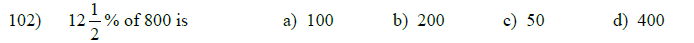103) Anju scored 39 marks out of 60 in Maths test. What % is this?

a) 75 % b) 35 % c) 60 % d) 65 %

104) What is the number whose 60 % is 90?

a) 450 b) 150 c) 100 d) 300

104) What is the number whose 60 % is 90?

a) 450 b) 150 c) 100 d) 300

105) What % of 75 minutes is 15 minutes?

a) 25 % b) 15 % c) 10 % d) 20 %

106) Ramesh scored 75 % in English Test. The marks were out of 40. How many marks did he score?

a) 30 b) 15 c) 35 d) 60

107) To change percentage into decimal, ________ by 100.

a) multiply b) divide c) add d) subtract

108) 8 % = ______

a) 0.8 b) 0.08 c) 0.008 d) 8.00

109) 2 ml = ________ % of a litre

a) 0.25 b) 20 c) 2.0 d) 0.2

110) 0.9 = ______

a) 9 % b) 90 % c) 900 % d) 9000 %

111) 25 % = _______

a)25/1000

b)25/10

c)25/100

d)2.5/100

112) 27.5 % kg is ______g

a) 27.5 g b) 275 g c) 2.75 g d) 0.275 g

113) In a class of 60 students, 55 % are girls. How many students are boys?

a) 27 b) 33 c) 45 d) 55

114) What % is 7.5 of 30?

a) 25 % b) 20 % c) 75 % d) 50 %

115) To convert fraction into percentage, multiply by

a) 10 b) 1000 c)100 d) 50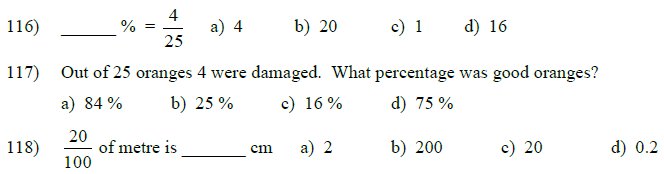119) 6 hundredths = _____ %

a) 6 b) 60 c) 0.6 d) 600

120) 25 % of 30 kg is = ______

a) 7 kg b) 8.5 kg c) 6.5 kg d) 7.5 kg

121) Which of the following is equivalent to 0.7 ?

a) 700 % b) 7 % c) 70 % d) 0.7 %

122) 63 paise as a percentage of a rupee = ____

a) 63 % b) 6.3% c) 0.63 % d) 6.03 %

123) The fraction in lowest term for 40 % = ____

a)3/5

b)4/5

c)1/5

d)2/5

124) What is the amount whose 25 % is Rs.75 ?

a) Rs.240 b) Rs. 18.75 c) Rs. 450 d) Rs.300

125) 30% of 300 = ____

a) 100 b) 90 c) 900 d) 10

126) Gain percentage = _______127) CP = Rs.145, Profit = Rs.15. Therefore SP is ________

a) Rs.106 b) Rs.120 c) Rs.160 d) Rs.150

128) CP = Rs.144, SP = Rs.168. Therefore profit is ________

a) Rs.14 b) Rs.24 c) Rs.42 d) Rs.312

129) If SP > CP then Profit =

a) SP – CP b) CP – SP c) CP + SP d)SP/CP

130) CP = Rs.69, SP = Rs.35, Overhead charges = Rs.10. Then the loss is _____

a) Rs.13 b) Rs.23 c) Rs.44 d) Rs.34

a) SP b) CP c) Profit d) Loss

132) A book is bought for Rs.20 and sold for Rs.16. The loss percentage is ______

a) 15 % b) 40 % c) 25 % d) 20 %

133) Profit = _______

a) CP – SP b) SP – CP c) SP + CP d) None of these

134) If the SP is less than the CP, there is ______

a) A profit b) No profit, No loss c) A gain d) A loss

135) Rohit bought an old bicycle for Rs.450 and spent Rs.85 on its repairing. He sold it for Rs.455.

Find the gain or loss. a) Profit = Rs.80 b) Loss = Rs.5 c) Loss = Rs.80 d) Loss = Rs.10

136) CP = Rs.300, Profit percentage = 20 % . Therefore the profit is _____

a) Rs.60 b) Rs.20 c) Rs.360 d) Rs.240

137) By selling a refrigerator for Rs. 5875, Mrs. Sara lost Rs.225. Find the price at which she bought the refrigerator.

a) Rs.5650 b) Rs.6100 c) Rs.5875 d) Rs.6000

138) A dozen eggs were bought for Rs.18 and sold at Rs.1.80 each. Find the profit or loss

a) Profit = Rs.3.60 b) Loss = Rs.3.60 c) Rs.19.80 d) Rs.16.20

139) Mohan bought a table for Rs. 485. He sold it at a profit of Rs. 60.75. Find the SP of the table.

a) Rs.424.25 b) Rs.545.25 c) Rs.424.75 d) Rs.545.75

140) The CP of a washing machine is Rs.7500 and the loss is Rs. 750 . What is the SP?

a) Rs.8250 b) Rs.6750 c) Rs.6570 d) Rs.8520

141) Mr. Sharma bought a cupboard for Rs.3200 and sold it for Rs.3104. Find his loss or profit.

a) Profit = Rs.96 b) Profit = Rs. 104 c) Loss = Rs. 104 d) Loss = Rs.96

142) Percentage of profit or loss is always calculated on ______

a) SP b) CP c) Overhead charges d) None of these

143) SP = Rs.218, Profit = Rs.12. Therefore CP = _____

a) Rs.206 b) Rs.230 c) Rs.106 d) Rs.236

144) A dealer sold a chair for Rs.90. He bought it for Rs.95 and spent Rs.20 on transportation. What was his profit/loss in this transaction?

a) Loss = Rs.25 b) Profit = Rs.25 c) Profit = Rs.15 d) Loss = Rs.15

145) Sam bought a book for Rs.428 and sold it sold it at a loss of Rs.68. What was the SP?

a) Rs.496 b) Rs.480 c) Rs.460 d) Rs.360

146) CP = Rs.100, SP = Rs.130, Overhead charges = Rs.20, then ,

a) Profit = Rs.50 b) Loss =Rs.10 c) Loss = Rs.50 d) Profit = Rs.10

147) A dozen pens were bought for Rs.120 and sold for Rs.15 each. Therefore the profit on each pen and the profit on all the pens is ______, ________ respectively.

a) Rs.5, Rs.60 b) Rs.10 , Rs.60 c) Rs.15, Rs.60 d) Rs.60, Rs.10

148) Excess amount in the Selling price as compared to the Cost price is called ________

a) Profit percentage b) Loss Percentage c) Loss d) Profit

149) CP = Rs.600, Profit percentage = 15% , then the profit is ______

a) Rs.510 b) Rs.90 c) Rs.15 d) Rs.85

150) CP = Rs.200, Loss percentage = 5 % , then the loss is ______

a) Rs.10 b) Rs.5 c) Rs. 25 d) Rs.20

151) A dot ( . ) represents a ______________

a) line b) point c) line segment d) stop

152) A ___________ has two end points.

a) line segment b) line c) ray d) point

153) A ray _______________

a) extends endlessly in two directions b) does not extend in any direction at all

c) extends endlessly in only one direction c) has two definite end points

154) A line segment _____________

a) has only one end point b) extends endlessly in only one direction

c) has no definite length d) has definite length

155) A right angle measures ________

a) exactly 90˚ b) less than 90˚ c) more than 90˚ d) 180˚

156) If the measures of two angles are same, they are called_______

a) complementary angles b) equal angles c) supplementary angles d) acute angles

157) ______________ measures more than 90˚ but less than 180˚

a) A right angle b) An acute angle c) An obtuse angle d) A straight angle

158) The measure of a straight angle is _________

a) 90˚ b) 0˚ c) 360˚ d) 180˚

159) A pair of angles are called supplementary to each other if the sum of their measures is ____

a) 180˚ b) 90˚ c) 90˚ minus the given angle d) 180˚ minus 90˚

160) A whole angle is an angle which measures ____

a) 90˚ b) 360˚ c) 180˚ d) 45˚

161) An angle measuring > 180˚ but less than 360˚ is called __________

a) supplementary angle b) equal angle c) reflex angle d) complementary angle

162) Two angles are called adjacent angles if they have ________

a) the same vertex b) they have equal measures

c) they lie in two separate figures d) they have different vertices

163) ________ pairs of vertically opposite angles are formed when two straight lines intersect at a point.

a) 3 b) 4 c) 2 d) No

164) A ___________ is used for measuring angles.

a) protractor b) compass c) divider d) ruler

165) The parts of an angle are ___________ and ___________

a) rays and lines b) arms and sides c) protractor and ruler d) arms and vertex

166) The distance around a circle is called the ____________

a) circumference b) area c) diameter d) radius

167) Diameter of a circle is __________

a) half of radius b) half of the circumference c) twice the radius d) one fourth of the circle

168) An arc is ________

a) any part of the radius b) any part of the diameter

c) any part of a chord d) any part of the circumference

a) a chord b) twice the diameter c) a part of an arc d) half of diameter

a) is a chord b) is not a chord c) is twice the diameter b) none of these

171) The distance between the centre and any point on the circle is called its _____.
a) circumference b) diameter c) chord d) radius

172) The diameter of a circle whose radius is 7 cm is
a) 21 cm
b) 44 cm
c) 3.5 cm
d) 14 cm

173) A Δ with all sides of different lengths is called
a) a scalene Δ b) an isosceles Δ c) an equilateral Δ d) a right angled Δ

174) A triangle is called ____________ triangle, if all its sides are equal.

a) like triangle b) equivalent triangle c) same triangle d) equilateral triangle

175) An obtuse angled triangle can have __________

a) one obtuse angle and two acute angles

b) one obtuse angle, one right angle and one acute angle

c) one obtuse angle and two right angles

d) two obtuse angles and one acute angle

176) A right angled triangle can have________

a) one right angle, one acute angle and one obtuse angle

b) one right angle and two obtuse angles

c) one obtuse angle and two right angles

d) one right angle and two acute angles

177) An isosceles triangle ____________

a) is a triangle whose two sides and two angles are equal

b) is a triangle whose all the three sides and three angles are equal

c) is a triangle whose all the three sides and angles measure different.

d) is a triangle with two obtuse angles.

178) Of the following cases, construction of which Δ is not possible?

a) 7 cm, 7 cm, 13 cm

b) 10 cm, 10 cm, 20 cm

c) 5 cm, 12 cm, 14 cm

d) 3 cm, 6 cm, 7 cm

179) Which one of the following triangles can be constructed?

a) 60°, 50°, 80°

b) 70°, 30°, 60°

c) 59°, 41°, 90°

d) 65°, 35°, 80°

180) The sum of the angles of a triangle is ______

a) equal to the measure of a straight angle b) equal to the measure of a right angle

c) greater than 180˚ d) less than 180˚

181) In triangle ABC, <A = 30˚, <B = 60˚ , then the triangle is________

a) an acute angled triangle b) an isosceles triangle

c) a right angledtriangle d) an equilateral triangle

182) The needles of a clock at 3.10 form ___________

a) an acute angle b) an obtuse angle c) a right angle d) a straight angle

183) A pentagon is a polygon with ______

a) 4 line segments b) 9 line segments c) 5 line segments d) 6 line segments

184) A polygon is a ________________

a) closed shape with any number of straight sides. b) closed shape with curved lines.

c) open shape with two straight sides. d) closed shape with both straight and curved sides.

185) ________, ________ and ________ are quadrilaterals.

a) Rectangle, triangle, pentagon b) Square, rectangle , triangle

c) Rectangle, Square, parallelogram d) Rectangle, parallelogram, octagon

186) Two lines are called parallel lines, if ________

a) they intersect at right angle

b) they meet at a common end point to form an angle

c) they do not intersect at any point and maintain the same distance between them all through

d) they form a straight angle

187) A good and common example for parallel lines is ____________

a) railway track b) spokes in a wheel

c) the protractor in the geometry box d) the compass in the geometry box

188) In which of the following group, all figures are quadrilaterals?

a) triangle, hexagon , pentagon and rectangle

b) rhombus, parallelogram, trapezium and rectangle

c) octagon, rhombus, rectangle and triangle

d) triangle, square, rectangle and rhombus

189) The sum of all the angles of a quadrilateral is ______

a) 360˚ b) 180˚ c) 90˚ d) 120˚

190) We join ____________ points to draw a triangle.

a) 3 collinear b) 3 Non collinear c) both a) and b) d) 2 collinear